# Computation on Graphs Graphs and Sparse Matrices Sparse

• Slides: 21
Download presentationComputation on Graphs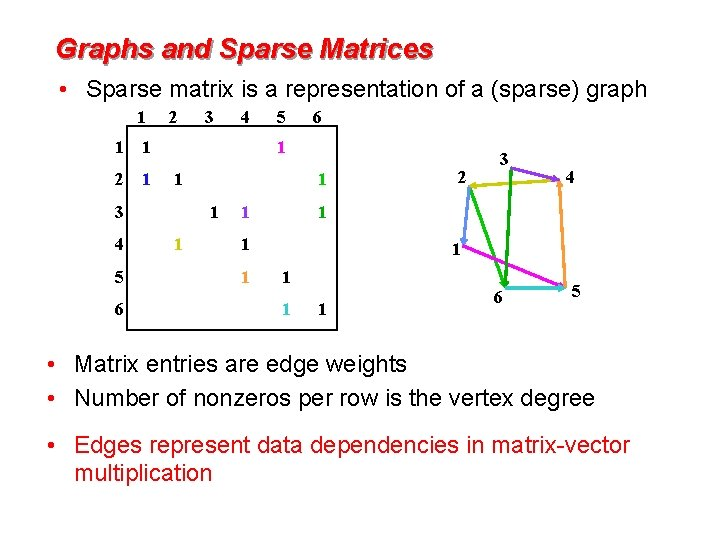Graphs and Sparse Matrices • Sparse matrix is a representation of a (sparse) graph 1 1 2 6 5 6 1 1 3 5 4 1 2 1 4 3 1 1 4 1 1 1 2 3 1 1 6 5 • Matrix entries are edge weights • Number of nonzeros per row is the vertex degree • Edges represent data dependencies in matrix-vector multiplication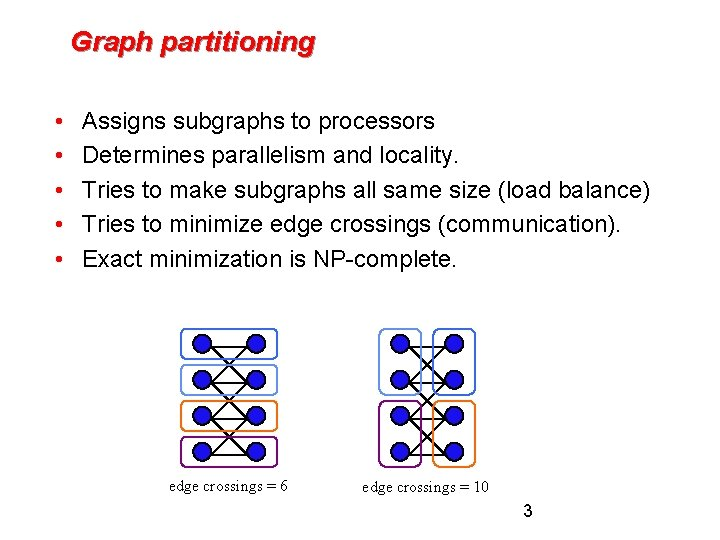Graph partitioning • • • Assigns subgraphs to processors Determines parallelism and locality. Tries to make subgraphs all same size (load balance) Tries to minimize edge crossings (communication). Exact minimization is NP-complete. edge crossings = 6 edge crossings = 10 3Link analysis of the web 1 1 2 2 3 4 1 2 3 4 7 5 4 5 6 3 6 7 • Web page = vertex • Link = directed edge • Link matrix: Aij = 1 if page i links to page j 5 6 7Web graph: Page. Rank (Google) [Brin, Page] An important page is one that many important pages point to. • Markov process: follow a random link most of the time; otherwise, go to any page at random. • Importance = stationary distribution of Markov process. • Transition matrix is p*A + (1 -p)*ones(size(A)), scaled so each column sums to 1. • Importance of page i is the i-th entry in the principal eigenvector of the transition matrix. • But the matrix is 1, 000, 000 by 1, 000, 000.A Page Rank Matrix • Importance ranking of web pages • Stationary distribution of a Markov chain • Power method: matvec and vector arithmetic • Matlab*P page ranking demo (from SC’ 03) on a web crawl of mit. edu (170, 000 pages)Social Network Analysis in Matlab: 1993 Co-author graph from 1993 Householder symposiumSocial Network Analysis in Matlab: 1993 Sparse Adjacency Matrix Which author has the most collaborators? >>[count, author] = max(sum(A)) count = 32 author = 1 >>name(author, : ) ans = Golub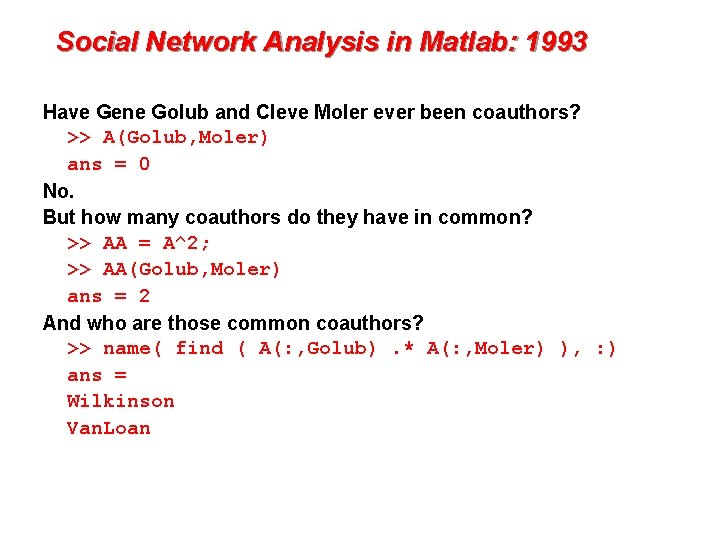Social Network Analysis in Matlab: 1993 Have Gene Golub and Cleve Moler ever been coauthors? >> A(Golub, Moler) ans = 0 No. But how many coauthors do they have in common? >> AA = A^2; >> AA(Golub, Moler) ans = 2 And who are those common coauthors? >> name( find ( A(: , Golub). * A(: , Moler) ), : ) ans = Wilkinson Van. Loan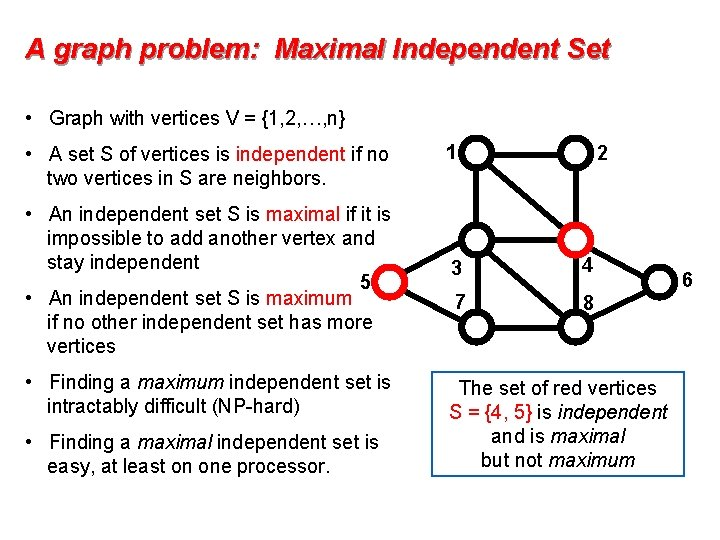A graph problem: Maximal Independent Set • Graph with vertices V = {1, 2, …, n} • A set S of vertices is independent if no two vertices in S are neighbors. • An independent set S is maximal if it is impossible to add another vertex and stay independent 5 • An independent set S is maximum if no other independent set has more vertices • Finding a maximum independent set is intractably difficult (NP-hard) • Finding a maximal independent set is easy, at least on one processor. 1 2 3 4 7 8 The set of red vertices S = {4, 5} is independent and is maximal but not maximum 6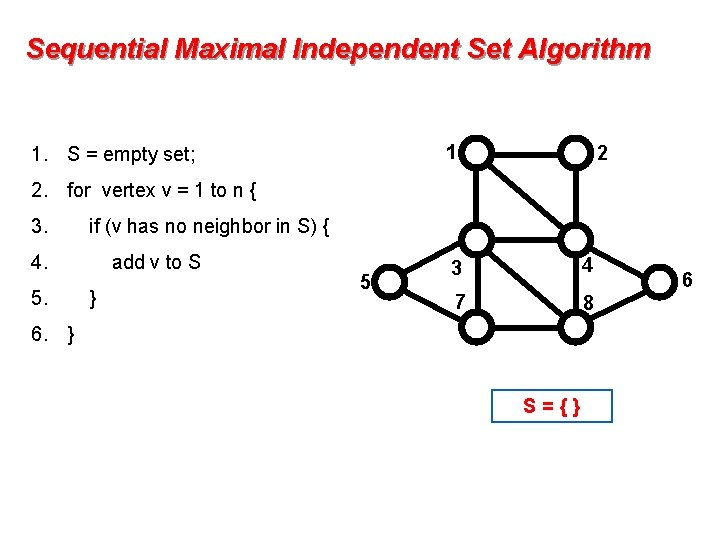Sequential Maximal Independent Set Algorithm 1 1. S = empty set; 2 2. for vertex v = 1 to n { 3. if (v has no neighbor in S) { 4. 5. add v to S } 5 3 4 7 8 6. } S={} 6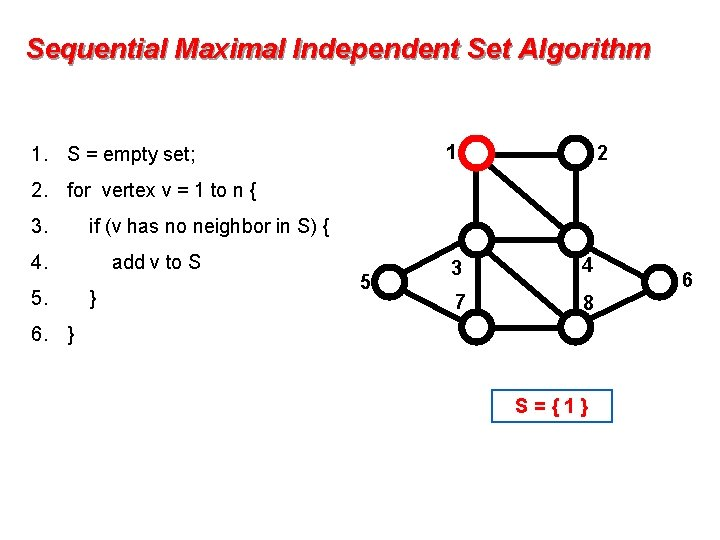Sequential Maximal Independent Set Algorithm 1 1. S = empty set; 2 2. for vertex v = 1 to n { 3. if (v has no neighbor in S) { 4. 5. add v to S } 5 3 4 7 8 6. } S={1} 6Sequential Maximal Independent Set Algorithm 1 1. S = empty set; 2 2. for vertex v = 1 to n { 3. if (v has no neighbor in S) { 4. 5. add v to S } 5 3 4 7 8 6. } S = { 1, 5 } 6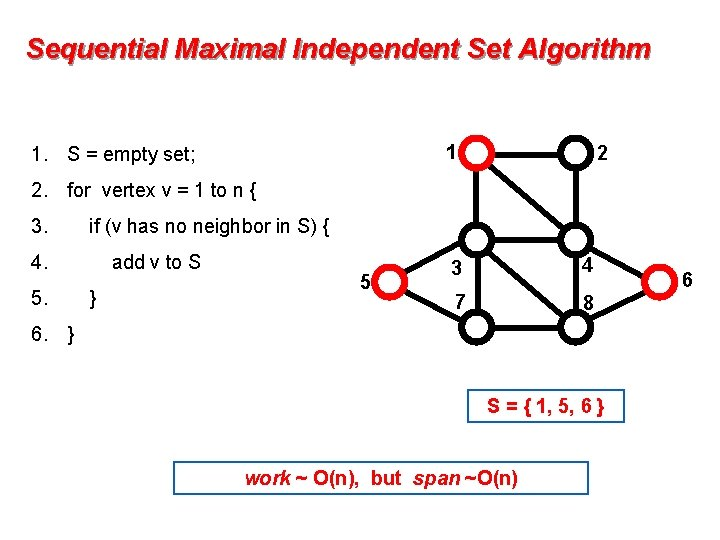Sequential Maximal Independent Set Algorithm 1 1. S = empty set; 2 2. for vertex v = 1 to n { 3. if (v has no neighbor in S) { 4. 5. add v to S } 5 3 4 7 8 6. } S = { 1, 5, 6 } work ~ O(n), but span ~O(n) 6Parallel, Randomized MIS Algorithm 1. S = empty set; C = V; [Luby] 1 2 2. while C is not empty { 3. label each v in C with a random r(v); 4. for all v in C in parallel { 5 if r(v) < min( r(neighbors of v) ) { 5. 6. move v from C to S; 7. remove neighbors of v from C; 8. 9. 10. } } } 3 4 7 8 S={} C = { 1, 2, 3, 4, 5, 6, 7, 8 } 6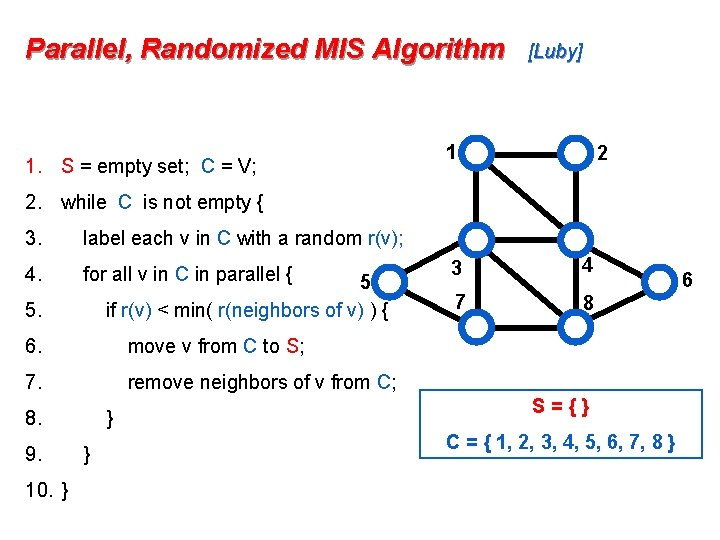Parallel, Randomized MIS Algorithm 1. S = empty set; C = V; [Luby] 1 2 2. while C is not empty { 3. label each v in C with a random r(v); 4. for all v in C in parallel { 5 if r(v) < min( r(neighbors of v) ) { 5. 6. move v from C to S; 7. remove neighbors of v from C; 8. 9. 10. } } } 3 4 7 8 S={} C = { 1, 2, 3, 4, 5, 6, 7, 8 } 6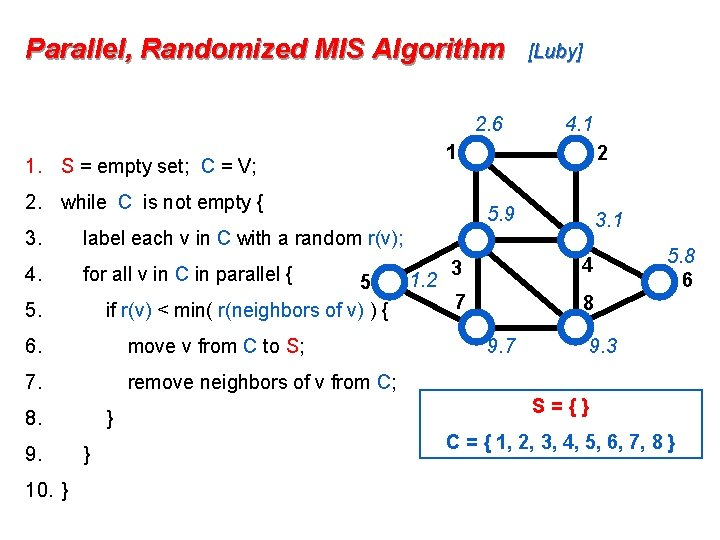Parallel, Randomized MIS Algorithm 2. 6 2. while C is not empty { 4. for all v in C in parallel { 5 if r(v) < min( r(neighbors of v) ) { 6. move v from C to S; 7. remove neighbors of v from C; 8. 10. } 2 5. 9 3. 1 label each v in C with a random r(v); 5. 9. 4. 1 1 1. S = empty set; C = V; 3. [Luby] } } 1. 2 3 4 7 8 9. 7 5. 8 6 9. 3 S={} C = { 1, 2, 3, 4, 5, 6, 7, 8 }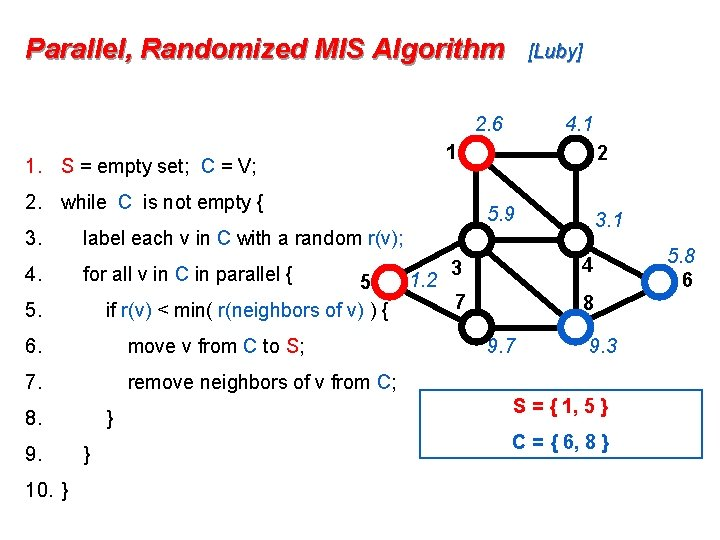Parallel, Randomized MIS Algorithm [Luby] 2. 6 1 1. S = empty set; C = V; 2. while C is not empty { 3. 4. for all v in C in parallel { 5 if r(v) < min( r(neighbors of v) ) { 6. move v from C to S; 7. remove neighbors of v from C; 8. 10. } 2 5. 9 3. 1 label each v in C with a random r(v); 5. 9. 4. 1 } } 1. 2 3 4 7 8 9. 7 9. 3 S = { 1, 5 } C = { 6, 8 } 5. 8 6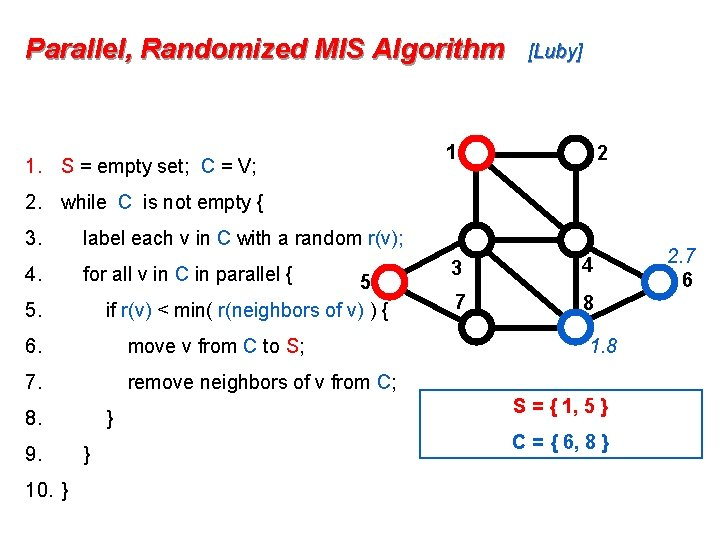Parallel, Randomized MIS Algorithm 1. S = empty set; C = V; [Luby] 1 2 2. while C is not empty { 3. 4. label each v in C with a random r(v); for all v in C in parallel { 5 if r(v) < min( r(neighbors of v) ) { 5. 6. move v from C to S; 7. remove neighbors of v from C; 8. 9. 10. } } } 3 4 7 8 1. 8 S = { 1, 5 } C = { 6, 8 } 2. 7 6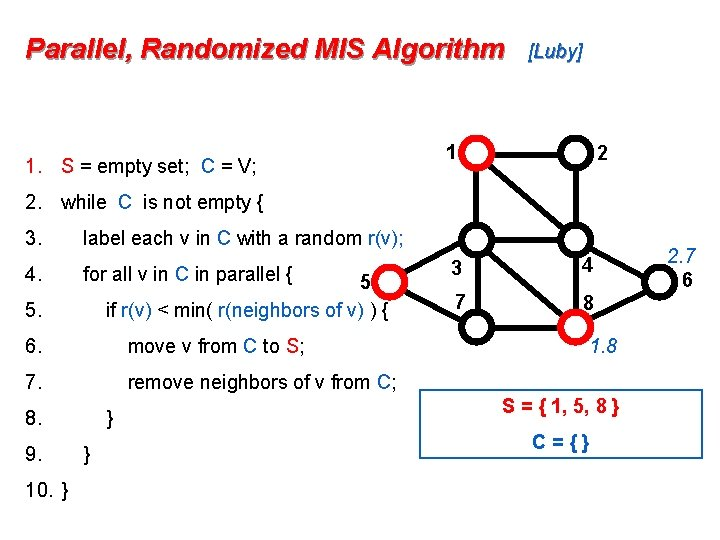Parallel, Randomized MIS Algorithm 1. S = empty set; C = V; [Luby] 1 2 2. while C is not empty { 3. 4. label each v in C with a random r(v); for all v in C in parallel { 5 if r(v) < min( r(neighbors of v) ) { 5. 6. move v from C to S; 7. remove neighbors of v from C; 8. 9. 10. } } } 3 4 7 8 1. 8 S = { 1, 5, 8 } C={} 2. 7 6Parallel, Randomized MIS Algorithm 1. S = empty set; C = V; [Luby] 1 2 2. while C is not empty { 3. label each v in C with a random r(v); 4. for all v in C in parallel { 5 if r(v) < min( r(neighbors of v) ) { 5. 6. move v from C to S; 7. remove neighbors of v from C; 8. 9. 10. } } } 3 4 7 8 Theorem: This algorithm “very probably” finishes within O(log n) rounds. work ~ O(n log n), but span ~O(log n) 6